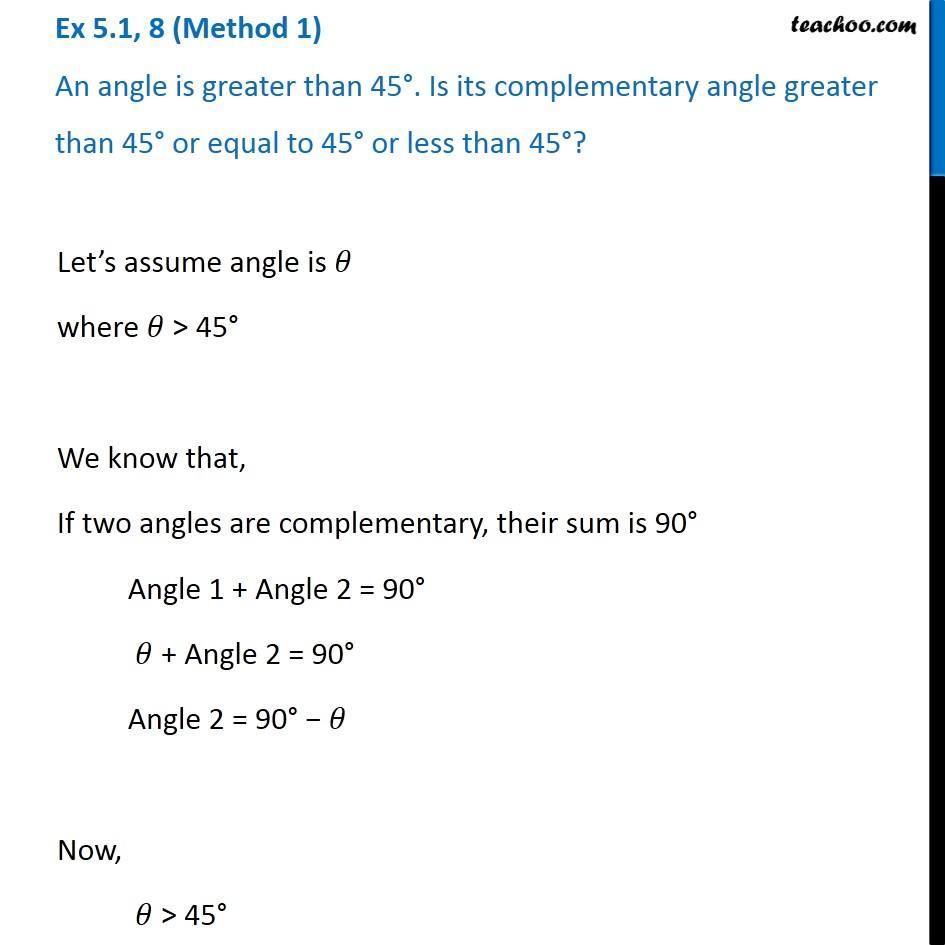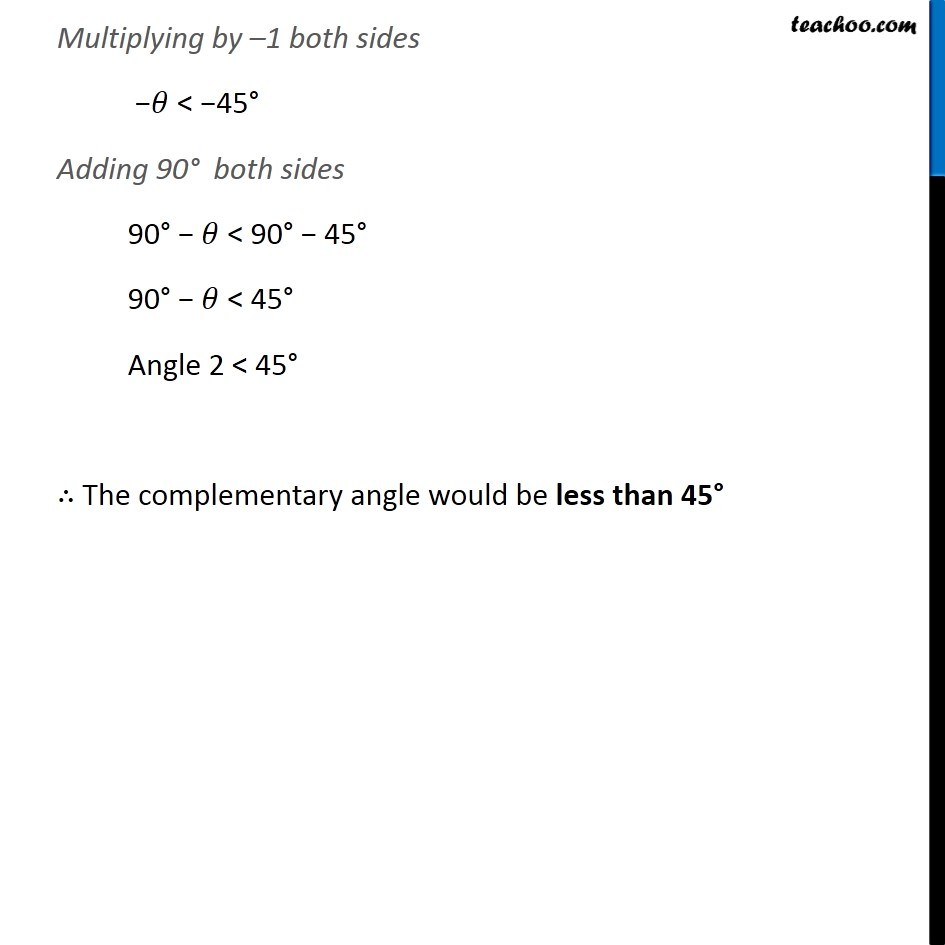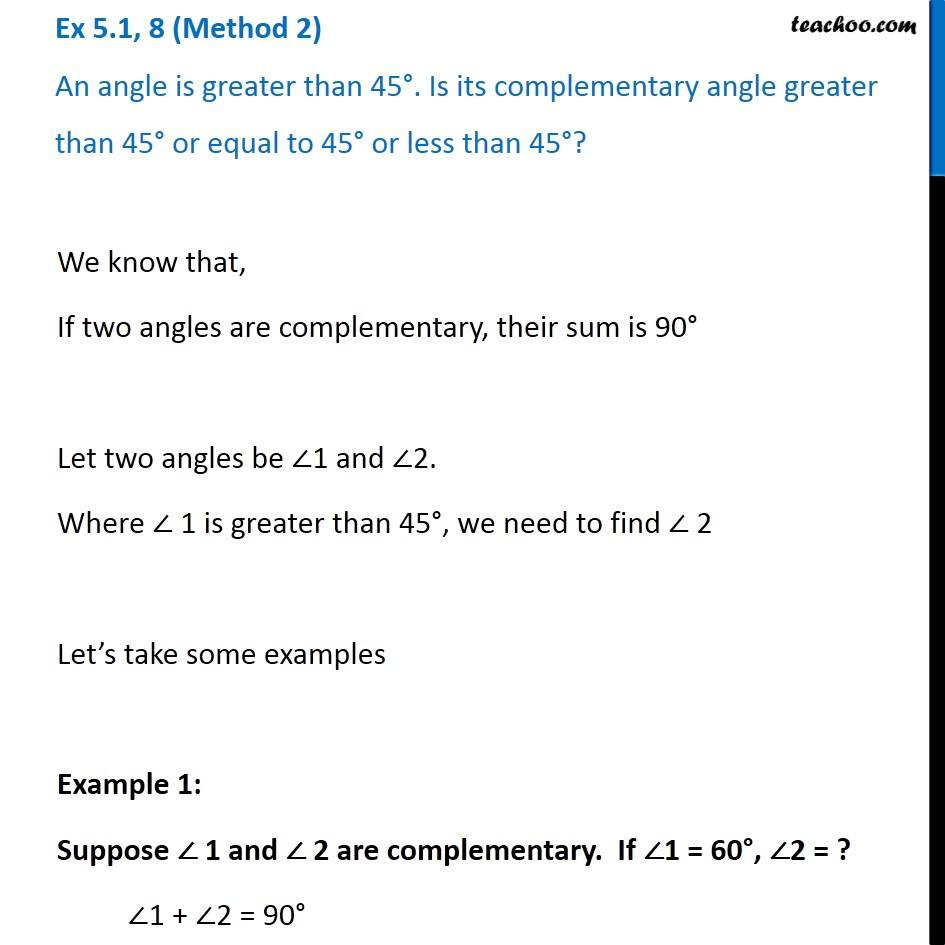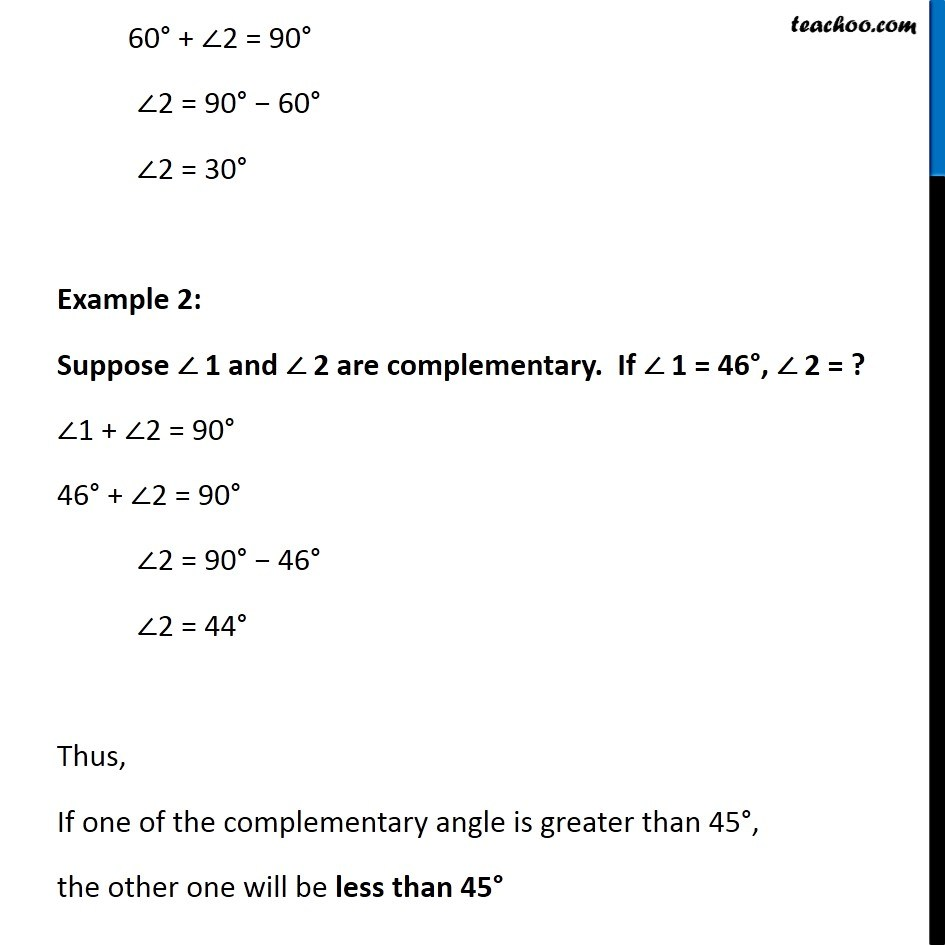Ex 5.1

Chapter 5 Class 7 Lines and Angles
Serial order wise### Transcript

Ex 5.1, 8 (Method 1) An angle is greater than 45°. Is its complementary angle greater than 45° or equal to 45° or less than 45°? Let’s assume angle is 𝜃 where 𝜃 > 45° We know that, If two angles are complementary, their sum is 90° Angle 1 + Angle 2 = 90° 𝜃 + Angle 2 = 90° Angle 2 = 90° − 𝜃 Now, 𝜃 > 45° Multiplying by –1 both sides −𝜃 < −45° Adding 90° both sides 90° − 𝜃 < 90° − 45° 90° − 𝜃 < 45° Angle 2 < 45° ∴ The complementary angle would be less than 45° Ex 5.1, 8 (Method 2) An angle is greater than 45°. Is its complementary angle greater than 45° or equal to 45° or less than 45°? We know that, If two angles are complementary, their sum is 90° Let two angles be ∠1 and ∠2. Where ∠ 1 is greater than 45°, we need to find ∠ 2 Let’s take some examples Example 1: Suppose ∠ 1 and ∠ 2 are complementary. If ∠1 = 60°, ∠2 = ? ∠1 + ∠2 = 90° 60° + ∠2 = 90° ∠2 = 90° − 60° ∠2 = 30° Example 2: Suppose ∠ 1 and ∠ 2 are complementary. If ∠ 1 = 46°, ∠ 2 = ? ∠1 + ∠2 = 90° 46° + ∠2 = 90° ∠2 = 90° − 46° ∠2 = 44° Thus, If one of the complementary angle is greater than 45°, the other one will be less than 45°